`mailto:` blog -at- heyrick -dot- eu

## Tassimo hacking - ITF-6 barcodes

Okay... So if I'm going to try a variety of different barcodes in the Tassimo, I'm going to need a better way of generating barcodes than getting PNGs from a website.

Which means, education time!

The first step is to know what our encoded number is to be. This is a five digit decimal number followed by a check digit. The five digits correspond to some sort of bitmap in the machine. Purpose unknown, that's why we're hacking. ☺
For the sake of argument, we shall use the five digits "03287". These are the digits that I use in my machine to make tea. The coffee maker thinks it is making endless cups of tea...actually it is, but not via capsule. It's making hot water so I can brew a nice Tetley.

Anyway - the digits are 03287.

### Creating the check digit

In order to create the check digit, we need to add together the values of the odd digits. In this case `0 + 2 + 7` which gives us `9`.
This value is then multiplied by three, giving `27`.

Next, we add in the even digits, or `3 + 8` giving us `11` which when added to `27` is a grand total of `38`.

The next calculation is to obtain the modulus of this value and ten. Essentially we are clipping off the first digit, so `38` becomes `38` or just `8`.

Finally, if the result of that was zero, then the check digit is zero.
However, if the result was not zero, then the check digit is ten minus the result. In this case it would be `10 - 8` giving us a check digit of `2`.

Which means our final code with check digit added is: `032872`.

### Interleaved two of five

ITF, when referring to barcodes (and not the Internet Task Force (wrong! it's actually the IETF), or International Tree Foundation, etc etc) stands for Interleaved Two of Five.

I'll explain what this means. It's actually quite clever.

As you will have noticed, traditional barcodes contain two primary parts. A bunch of lines of various widths. and a bunch of spaces between the lines, also of different widths.

Actually, in ITF, the lines are only two widths - a thin and a thick. The thick is defined as 2-3 times the width of the thin. This is a simpler arrangement than, say, EAN product barcodes (go find a book, can of coke, etc) which appear (visually, not looked it up) to have three widths).
In ITF, just as there are thin lines and thick lines, there can be thin spaces and thick spaces.

Numbers are dealt with in pairs. The first number is represented in the lines using exactly five lines. Exactly two of these lines are thick. This is why it is called "two of five". The arrangement of thick/thin determines the number.
Now here's the clever part. The second number is represented in the spaces, using exactly five spaces, and as for the lines, two will be thick spaces and two will be thin.

The arrangement of lines/spaces to number is as follows, with '0' meaning thin and '1' meaning thick:

```0 is 0, 0, 1, 1, 0
1 is 1, 0, 0, 0, 1
2 is 0, 1, 0, 0, 1
3 is 1, 1, 0, 0, 0
4 is 0, 0, 1, 0, 1
5 is 1, 0, 1, 0, 0
6 is 0, 1, 1, 0, 0
7 is 0, 0, 0, 1, 1
8 is 1, 0, 0, 1, 0
9 is 0, 1, 0, 1, 0```

Our first two digits are zero and three. This means that we want our lines to be thin, thin, thick, thick, thin and we want our spaces to be thick, thick, thin, thin, thin.

If we can draw a rubbish bit of barcode in ASCII, that would look like this:

```##    ##    ####  ####  ##
##    ##    ####  ####  ##
##    ##    ####  ####  ##
##    ##    ####  ####  ##  ```

If we add the run-in, which is two thin lines with two thin spaces, this becomes:

```##  ##  ##    ##    ####  ####  ##
##  ##  ##    ##    ####  ####  ##
##  ##  ##    ##    ####  ####  ##
##  ##  ##    ##    ####  ####  ##  ```

Compare this against the actual barcode used in the coffee maker: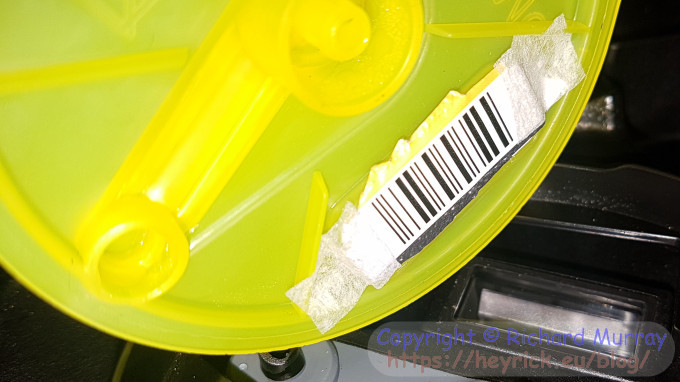You can see it clearly, reading left to right: thin line and thin space, twice. Followed by thin line, big space, thin line, big space, thick line, thin space, thick line, thin space, thin line, thin space.

We now simply repeat this for the third and fourth digits, and once more again for the fifth and sixth digits.

Afterwards, the run-out code of a thick line, thin space, thin line is added.

And, voilà, we have just created ourselves a valid ITF barcode.

Here's the result of my first program to do this:

```*ITFgen
Enter numeric code (5 digits) : ?03287
Check digit is 2
Therefore the full code is 032872

Generated barcode:
##  ##  ##    ##    ####  ####  ##  ##    ####  ##  ##    ####  ##  ##    ##  ####  ####    ####  ##
##  ##  ##    ##    ####  ####  ##  ##    ####  ##  ##    ####  ##  ##    ##  ####  ####    ####  ##
##  ##  ##    ##    ####  ####  ##  ##    ####  ##  ##    ####  ##  ##    ##  ####  ####    ####  ##
##  ##  ##    ##    ####  ####  ##  ##    ####  ##  ##    ####  ##  ##    ##  ####  ####    ####  ##
##  ##  ##    ##    ####  ####  ##  ##    ####  ##  ##    ####  ##  ##    ##  ####  ####    ####  ##
##  ##  ##    ##    ####  ####  ##  ##    ####  ##  ##    ####  ##  ##    ##  ####  ####    ####  ##
##  ##  ##    ##    ####  ####  ##  ##    ####  ##  ##    ####  ##  ##    ##  ####  ####    ####  ##
##  ##  ##    ##    ####  ####  ##  ##    ####  ##  ##    ####  ##  ##    ##  ####  ####    ####  ##
##  ##  ##    ##    ####  ####  ##  ##    ####  ##  ##    ####  ##  ##    ##  ####  ####    ####  ##
##  ##  ##    ##    ####  ####  ##  ##    ####  ##  ##    ####  ##  ##    ##  ####  ####    ####  ##
##  ##  ##    ##    ####  ####  ##  ##    ####  ##  ##    ####  ##  ##    ##  ####  ####    ####  ##
##  ##  ##    ##    ####  ####  ##  ##    ####  ##  ##    ####  ##  ##    ##  ####  ####    ####  ##

Done.
*```

Zooming this image up 800% in NetSurf and moving the TaskWindow just underneath matched up the ASCII art barcode to the real thing.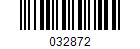Perfect match.

### Making this a useful barcode

First we need to work out how wide our barcode is going to be.

The run-in is two thin lines and two thin spaces. Let's count thin as '1' and thick as '2'. So the run-in gives us `4`. The run-out is a thick line, thin space, thin line. This also gives us a value of `4`.
Now we know that each number is comprised of two thick lines and three thin lines. `2+2+1+1+1` is `7`. Double that because the second number is in the spaces, encoded the same way. So a number pair is a width of `14`.

Run-in (`4`), three number pairs (`42`), and run-out (`4`) gives us a raw barcode width of fifty elements.

Here we go...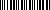Okay, it's correct but it's a bit rubbish. It would make a lot more sense to have an image that we can work with. So simply multiply everything by four. A width of 200 pixels instead of fifty.
It'll look like this: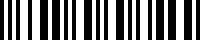### Creating the sprite

It's not so difficult to turn this into something to create a barcode graphic. What I do is work out what the barcode encoding should be, and write this across the top line of the sprite (line 49, as line zero is the bottom).
Once the encoding has been written, and remember we're writing four pixels for every element across, I will go back and scan each pixel in the top line and copy it to all the other lines.

Doing this, our program when run now looks like this:

```*ITFgen
Enter numeric code (5 digits) : ?03287
Check digit is 2; full code is 032872
Creating sprite...generating barcode...replicating...saving as "032872".
*```
and the resultant sprite is this: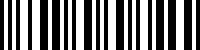It's not the same as the previous sprite. This one was generated programmatically, the previous one was created by hand in Paint (because at that time, ITFgen didn't create sprites).

### ITFgen

Here's a listing of the software. It isn't designed to be efficient, it's designed to get a job done and be readable.

```REM >ITFgen
REM
REM by Rick Murray
REM
REM Friday, 10th August 2018

RESTORE +0
DIM bars%(10, 5)
FOR l% = 0 TO 9
READ bars%(l%, 1), bars%(l%, 2), bars%(l%, 3), bars%(l%, 4), bars%(l%, 5)
NEXT

REM Get the code
INPUT "Enter numeric code (5 digits) : ";code\$

REM Validate
IF (LEN(code\$) <> 5) THEN PRINT "Incorrect length." : END
FOR l% = 1 TO 5
a% = (ASC(MID\$(code\$, l%, 1)) - 48)
IF ((a% < 0) OR (a% > 9)) THEN PRINT "Numbers only." : END
NEXT

REM Work out the check digit
a% = (ASC(MID\$(code\$, 1, 1)) - 48)
b% = (ASC(MID\$(code\$, 2, 1)) - 48)
c% = (ASC(MID\$(code\$, 3, 1)) - 48)
d% = (ASC(MID\$(code\$, 4, 1)) - 48)
e% = (ASC(MID\$(code\$, 5, 1)) - 48)
f% = ((a% + c% + e%) * 3) + (b% + d%)
f% = f% MOD 10
IF (f% = 0) THEN
check% = 0
ELSE
check% = 10 - f%
ENDIF
code\$ = code\$ + STR\$(check%)
PRINT "Check digit is "+STR\$(check%)+"; full code is "+code\$

REM Create the sprite
PRINT "Creating sprite...";
DIM spr% 2048 : REM Size is 200x50 at 1bpp, or 1250 bytes, plus header
FOR loop% = 0 TO 2047 STEP 4 : spr%!loop% = 0 : NEXT : REM Clear to white
spr%!0 = 2048
spr%!8 = 16
SYS "OS_SpriteOp", (9 + 256), spr%
SYS "OS_SpriteOp", (15 + 256), spr%, code\$, 0, 200, 50, (1<<27)+(90<<14)+(90<<1)+1
REM                                                     1bpp,   y=90dpi, x=90dpi
pos% = 0

REM Run-in: Narrow bar, narrow space, narrow bar, narrow space
PRINT "generating barcode...";

REM Now generate the code
FOR loop% = 1 TO 5 STEP 2
REM Get our two digits
first%  = (ASC(MID\$(code\$, loop%, 1)) - 48)
second% = (ASC(MID\$(code\$, (loop%+1), 1)) - 48)

REM Now step through the barcode data elements
FOR recurse% = 1 TO 5
line%  = bars%(first%, recurse%)  : REM Line
space% = bars%(second%, recurse%) : REM Space

IF (line% = 1) THEN
ELSE
ENDIF

IF (space% = 1) THEN
ELSE
ENDIF
NEXT
NEXT

REM Run-out: Wide bar, narrow space, narrow bar

REM That was across line 49. So now replicate to fill the sprite.
PRINT "replicating...";
FOR pos% = 0 TO 199
SYS "OS_SpriteOp", (41 + 256), spr%, code\$, pos%, 49 TO ,,,,,col%,tint%
FOR loop% = 0 TO 48
SYS "OS_SpriteOp", (42 + 256), spr%, code\$, pos%, loop%, col%, tint%
NEXT
NEXT

REM Save the sprite
PRINT "saving as """+code\$+"""."
SYS "OS_SpriteOp", (12 + 256), spr%, code\$

END

REM Barcode data (0-9)
DATA 0, 0, 1, 1, 0
DATA 1, 0, 0, 0, 1
DATA 0, 1, 0, 0, 1
DATA 1, 1, 0, 0, 0
DATA 0, 0, 1, 0, 1
DATA 1, 0, 1, 0, 0
DATA 0, 1, 1, 0, 0
DATA 0, 0, 0, 1, 1
DATA 1, 0, 0, 1, 0
DATA 0, 1, 0, 1, 0

LOCAL skip%, count%

IF (thick% = 1) THEN count% = 8 ELSE count% = 4

FOR skip% = 1 TO count%
SYS "OS_SpriteOp", (42 + 256), spr%, code\$, pos%, 49, 1, 0
pos% = pos% + 1
NEXT
ENDPROC

LOCAL count%

IF (thick% = 1) THEN count% = 8 ELSE count% = 4
pos% = pos% + count% : REM No need to set pixels to white!
ENDPROC```

Now we not only know how ITF barcodes are created, we also have software that we can use to create suitable barcodes.

Here's the software as a zip file: itfgen.zip (!.6KiB)

All of this reminds me... Time for a spot of tea.

Please note that while I check this page every so often, I am not able to control what users write; therefore I disclaim all liability for unpleasant and/or infringing and/or defamatory material. Undesired content will be removed as soon as it is noticed. By leaving a comment, you agree not to post material that is illegal or in bad taste, and you should be aware that the time and your IP address are both recorded, should it be necessary to find out who you are. Oh, and don't bother trying to inline HTML. I'm not that stupid! ☺ ADDING COMMENTS DOES NOT WORK IF READING TRANSLATED VERSIONS.

You can now follow comment additions with the comment RSS feed. This is distinct from the b.log RSS feed, so you can subscribe to one or both as you wish.

 David Pilling, 15th August 2018, 18:52 Gratuitous mention of the bar code generation in Ovation Pro - which uses a bar code font. ISTR Alan Gibson, Liquid Silicon, specialised in bar codes for RISC OS. Thomas, 15th June 2021, 00:45 Can u pls tell me where to execute that ITFgen? Would love to have it for Linux :) Rick, 15th June 2021, 07:28 It is written in BBC BASIC that runs on RISC OS.  The SpriteOp calls create a bitmap, and then set pixels within, so it could be ported to C... Claudiu, 26th December 2022, 14:31 Hi:) i'm so very late to the party but i figured i might ask, maybe i get lucky. I use windows 11 and i have tried to install BBC Basic, but running the code comes back with an error: "Creating sprite... No such system call " at the SYS"Os_SpriteOp" line. Is there any other way to run it please? or a win version ?  thank you !! Rick, 26th December 2022, 15:52 Sorry, the SYS commands make OS calls, so if BASIC for Windows doesn't support sprites, it won't work.  As a very basic hack, try this.  REM out all of the SpriteOp calls. Also REM out the "`DIM savearea% sasize%`" or it'll error.  At the top where it says creating sprite, insert this: ```MODE 28 GCOL 255, 255, 255 : REM White RECTANGLE FILL 0, 0, 460, 100``` Change the line before `PRINT \$binary%` to say: `MOVE 92, 14` Now finally modify `DEFPROCaddbar()` so the middle part says this: ```FOR skip% = 1 TO count% GCOL 0, 0, 0 : REM Black FOR row% = 32 TO 180 POINT (pos% << 1) + 30, row% NEXT pos% = pos% + 1 NEXT``` That ought to draw the barcode on the screen, so you can take a snapshot or whatever to capture it.  You may want to look at the BBfW help to see if it provides it's own sprite/icon handling, perhaps using the Windows GDI? It'll be simpler if it does.  Alternatively, you could try installing RPCEmu (Google it) and running a copy of RISC OS under emulation?  If you know another language, such as PHP or Python, the code should be pretty easy to translate. It's not a difficult algorithm. ;) Claudiu, 26th December 2022, 17:36 Wow thank you for the fast response !!!  I REM'ed out all the SYS "OS_SpriteOp". I can not find DIM savearea% sasize% - there is only one DIM but has something else after I can not find the PRINT \$binary%.  I know i'm asking for more, but would you be so kind to post the entire code with the hack so i can copy/paste in BBC Basic ? I am VERY good at copy/paste ...  Thank you !!! Rick, 26th December 2022, 18:00 Try this. If it doesn't work, I'm afraid you're on your own as it works fine on RISC OS. It ought to behave similarly with BBfW... ```REM >ITFgenPix REM REM by Rick Murray REM REM Monday, 26th December 2022 ON ERROR PRINT REPORT\$+" at "+STR\$(ERL) : END RESTORE +0 DIM bars%(10, 5) FOR l% = 0 TO 9 READ bars%(l%, 1), bars%(l%, 2), bars%(l%, 3), bars%(l%, 4), bars%(l%, 5) NEXT REM Get the code MODE 28 INPUT "Enter numeric code (5 digits) : ";code\$ REM Validate IF (LEN(code\$) <> 5) THEN PRINT "Incorrect length." : END FOR l% = 1 TO 5 a% = (ASC(MID\$(code\$, l%, 1)) - 48) IF ((a% < 0) OR (a% > 9)) THEN PRINT "Numbers only." : END NEXT REM Work out what this code is in binary (for the annotation) DIM binary% 17 SYS "XOS_ConvertBinary2", VAL(code\$), binary%, 17 TO , t% t%?0 = 13 REM Work out the check digit a% = (ASC(MID\$(code\$, 1, 1)) - 48) b% = (ASC(MID\$(code\$, 2, 1)) - 48) c% = (ASC(MID\$(code\$, 3, 1)) - 48) d% = (ASC(MID\$(code\$, 4, 1)) - 48) e% = (ASC(MID\$(code\$, 5, 1)) - 48) f% = ((a% + c% + e%) * 3) + (b% + d%) f% = f% MOD 10 IF (f% = 0) THEN check% = 0 ELSE check% = 10 - f% ENDIF code\$ = code\$ + STR\$(check%) PRINT "Check digit is "+STR\$(check%)+"; full code is "+code\$ REM Setup screen GCOL 255,255,255 RECTANGLE FILL 0, 0, 460, 100 pos% = 0 REM Run-in: Narrow bar, narrow space, narrow bar, narrow space PROCaddbar(0) PROCaddspace(0) PROCaddbar(0) PROCaddspace(0) REM Now generate the rest of the barcode FOR loop% = 1 TO 5 STEP 2 REM Get our two digits first% = (ASC(MID\$(code\$, loop%, 1)) - 48) second% = (ASC(MID\$(code\$, (loop%+1), 1)) - 48) REM Now step through the barcode data elements FOR recurse% = 1 TO 5 line% = bars%(first%, recurse%) : REM Line space% = bars%(second%, recurse%) : REM Space IF (line% = 1) THEN PROCaddbar(1) : REM Wide line ELSE PROCaddbar(0) : REM Narrow line ENDIF IF (space% = 1) THEN PROCaddspace(1) : REM Wide space ELSE PROCaddspace(0) : REM Narrow space ENDIF NEXT NEXT REM Run-out: Wide bar, narrow space, narrow bar PROCaddbar(1) PROCaddspace(0) PROCaddbar(0) REM Add the annotation text VDU 5 MOVE 28, 14 PRINT code\$+" "+\$binary% VDU 4 REM Done END REM Barcode data (0-9) DATA 0, 0, 1, 1, 0 DATA 1, 0, 0, 0, 1 DATA 0, 1, 0, 0, 1 DATA 1, 1, 0, 0, 0 DATA 0, 0, 1, 0, 1 DATA 1, 0, 1, 0, 0 DATA 0, 1, 1, 0, 0 DATA 0, 0, 0, 1, 1 DATA 1, 0, 0, 1, 0 DATA 0, 1, 0, 1, 0 DEFPROCaddbar(thick%) LOCAL skip%, count% IF (thick% = 1) THEN count% = 8 ELSE count% = 4 FOR skip% = 1 TO count% GCOL 0, 0, 0 FOR row% = 32 TO 180 POINT (pos%<<1) + 30, row% NEXT pos% = pos% + 1 NEXT ENDPROC DEFPROCaddspace(thick%) LOCAL count% IF (thick% = 1) THEN count% = 8 ELSE count% = 4 pos% = pos% + count% : REM No need to set pixels to white! ENDPROC``` Claudiu, 26th December 2022, 20:25 Unfortunatelly it does not work.  I went the other way: installed RPCEmu, managed to get the file onto it. But no idea how to run it. *Exec filename just spits out a lot of errors. At least i'm running it in the correct path.  All i wanted was a bar code for 200, 250, 300 and 400 ml of 80-90 degrees water and i ended up trying to run an OS i never heard of before.  Fun :) Well it is what it is:) My skills are not up for this unfortunatelly.  thank you very much for the guidance:) we tried at least. Rick, 26th December 2022, 21:22 Well, I'm impressed that you got as far as trying to *Exec it.  If the program is present as a text file (in other words you cut and pasted it there), from the command line you'll want to:  *BASIC TEXTLOAD "" RUN  That ought to do it. Rick, 26th December 2022, 21:23 By the way, what are the codes for the 80-90 degrees water in the various sizes? Claudiu, 26th December 2022, 21:56 Ahaha tyty :)  So this is how you run that text file. Great. It works perfectly with the original script and generates a sprite. Weird file extension and all, have to find out how to trabsfer that to a jpg or something. Meanwhile i just snip it from windows.  The modified script does not run, it complains about a line too long, but that doesn't matter now as the original one works.  about thr 80-90 degrees - no idea about the codes, that's how hot i'd like the water to be :)  I'm gonna take some codes you posted around here and try to print them to see how it goes. Claudiu, 26th December 2022, 22:44 Ha! i found DPScan that can open then ff9 files and save as jpg. I'll play around with it and post back results.  The reason i ressurected this old thread for an ancient coffee maker is that i had one tassimo machino in storage and i decided to repurpose it. Trying to avoid buying anything new these days. Money is hard to come by and inflation is well... through the roof

 Add a comment (v0.11) [help?] . . . try the comment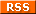feed! Your name Your email (optional) Validation Are you real? Please type 99411 backwards. Your comment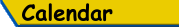Mon Tue Wed Thu Fri Sat Sun «   August 2018   » 1 2 3 4 5 6 7 8 9 10 11 12 13 14 15 16 17 18 19 20 21 22 23 24 25 26 27 28 29 30 31

(Felicity? Marte? Find out!)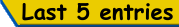List all b.log entries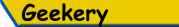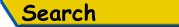Search Rick's b.log!
PS: Don't try to be clever.
It's a simple substring match.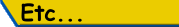Last read at 14:25 on 2023/12/04.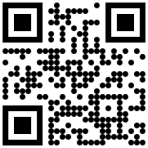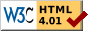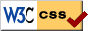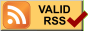© 2018 Rick Murray This web page is licenced for your personal, private, non-commercial use only. No automated processing by advertising systems is permitted. RIPA notice: No consent is given for interception of page transmission.

 Have you noticed the watermarks on pictures? Read the explanation.
Next entry - 2018/08/15